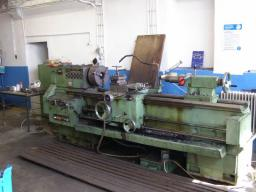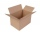# Lathe

From the cube of edge 37 cm was lathed maximum cylinder. What percentage of the cube is left as waste after lathed?

Correct result:

p =  21.5 %

#### Solution:

$p = 100\% \cdot \dfrac{ V_1-V_2}{ V_1} = 100\% \cdot \dfrac{ 37^3-\pi \dfrac{ 37^2}{4} \cdot 37 }{ 37^3} = 100 \cdot (1- \dfrac{ \pi}{4} ) = 21.5 \%$We would be very happy if you find an error in the example, spelling mistakes, or inaccuracies, and please send it to us. We thank you!Tips to related online calculators
Check out our ratio calculator.

## Next similar math problems:

• Cube into cylinderIf we dip a wooden cube into a barrel with a 40cm radius, the water will rise 10 cm. What is the size of the cube edge?
• Length of the edgeFind the length of the edge of a cube that has a cm2 surface and a volume in cm3 expressed by the same number.
• Calculate 3Calculate the cube volume whose edge is 3x-1,3x-1,3x-1
• Cuboid and ratioFind the dimensions of a cuboid having a volume of 810 cm3 if the lengths of its edges coming from the same vertex are in ratio 2: 3: 5
• EightEight small Christmas balls with a radius of 1 cm have the same volume as one large Christmas ball. What has a bigger surface: eight small balls, or one big ball?
• Cube surfce2volumeCalculate the volume of the cube if its surface is 150 cm2.
• Wall thicknessThe hollow metal ball has an outside diameter of 40 cm. Determine the wall thickness if the weight is 25 kg and the metal density is 8.45 g/cm3.
• The volume 2The volume of a cube is 27 cubic meters. Find the height of the cube.
• Cube diagonalsCalculate the length of the side and the diagonals of the cube with a volume of 27 cm3.
• Cardboard boxPeter had square cardboard. The length of the pages was an integer in decimetres. He cut four squares with a side of 3 dm from the corners and made a box out of it, which fit exactly 108 cubes with an edge 1 dm long. Julia cut four squares with a side of
• The ballThe ball has a radius of 2m. What percentage of the surface and volume is another sphere whose radius is 20% larger?
• Spherical segmentCalculate the volume of a spherical segment 18 cm high. The diameter of the lower base is 80 cm, the upper base 60 cm.
• Right circular coneThe volume of a right circular cone is 5 liters. Calculate the volume of the two parts into which the cone is divided by a plane parallel to the base, one-third of the way down from the vertex to the base.
• Magnified cubeIf the lengths of the edges of the cube are extended by 5 cm, its volume will increase by 485 cm3. Determine the surface of both the original and the magnified cube.
• Cuboid to cubeA cuboid with dimensions of 9 cm, 6 cm, and 4 cm has the same volume as a cube. Calculate the surface of this cube.
• Alien shipThe alien ship has the shape of a sphere with a radius of r = 3000m, and its crew needs the ship to carry the collected research material in a cuboid box with a square base. Determine the length of the base and (and height h) so that the box has the large
• Volume of ballFind the volume of a volleyball that has a radius of 4 1/2 decimeters. Use 22/7 for π